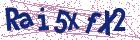# Application of modified simple equation method to Burgers, Huxley and Burgers-Huxley equations

Document Type : Research Article

Authors

Department of Engineering Sciences, Faculty of Technology and Engineering East of Guilan, University of Guilan, Rudsar-Vajargah, Iran.

Abstract

In this paper, modified simple equation method has been applied to ob-tain generalized solutions of Burgers, Huxley equations and combined forms of these equations. The new exact solutions of these equations have been obtained. It has been shown that the proposed method provides a very effective, and powerful mathematical tool for solving nonlinear partial differential
equations.

Keywords20.1001.1.24236977.2015.5.2.5.2

#### References

1. Biazar, J., Babolian, E., Nouri, A. and Islam, R. An alternate algorithm for computing Adomian Decomposition method in special cases, Applied Mathematics and Computation 38 (2003)523-529.
2. Burgers, M. The Nonlinear Diusion Equation, Reidel, Dordtrecht, 1974.
3. Duan, L. X. and Lu, Q. S. ursting oscillations near codimension-two bifurcations in the Chay Neuron model, International Journal of Nonlinear Sciences and Numerical Simulation 7(1) (2006) 59-64.
4. Ebaid, A. Exact solitary wave solutions for some nonlinear evolution equations via Exp-function method, Phys.Lett. A 365 (2007) 213-219.
5. Hariharan, G. and Kannan, K. Haar wavelet method for solving FitzHugh-Nagumo equation, International Journal of Mathematical and Statistical Sciences 2 (2) (2010) 59-63.
6. He, J.H. Application of homotopy perturbation method to nonlinear wave equations, Chaos Solitons Fractals 26 (2005) 695-700.
7. He, J. H. Modified Hodgkin-Huxley model, Chaos, Solitons,and Fractals 29(2) (2006) 303-306.
8. He, J. H. Resistance in cell membrane and nerve fiber, Neuroscience Letters 373(1) (2005) 48-50.
9. He, J. H. Variational iteration method for autonomous ordinary differential systems, Appl. Math. Comput. 114 (2000) 115-123.
10. He, J. H. Variational iteration method - a kind of non-linear analytical technique: some examples, Int. J. Nonlinear Mech. 34 (1999) 699-708.
11. He, J. H. and Wu, X. H. Exp-function method for nonlinear wave equations, Chaos Solitons Fractals, 30 (2006) 700-708.
12. He, J. H. and Wu, X. H. Modified Morris-Lecar model for interacting ion channels, Neurocomputing 64 (2005) 543-545.
13. Hodgkin, A. L. and Huxley, A. F. A quantitative description of membrane current and its application to conduction and excitation in nerve, The Journal of Physiology 117(4) (1952) 500-544.
14. Jawad, A. J. M., Petkovic, M. D. and Biswas, A. Modified simple equation method for nonlinear evolution equations, Appl. Math. Comput. 217 (2010) 869-877.
15. khan, K. and Akbar, M. A. Exact and solitary wave solutions for the Tzitzeica-Dodd-Bullough and the modified Kdv-Zakharov-Kuznetsov equations using the modified simple equation method, Ain Shams Engineering
Journal 4(4) (2013)903-909.
16. Kudryashov, N. A. Nadejda B. Loguinova, Extended simplest equation method for nonlinear differential equations, Applied Mathematics and Computation 205 (2008) 396-402.
17. Liao, S. J. The proposed homotopy analysis techniques for the solution of nonlinear problems, Ph.D. dissertation. Shanghai: Shanghai Jiao Tong,University; 1992 [in English].
18. Liu, S. Q., Fan, T. and Lu, Q. S. The spike order of the winnerless competition -WLC-model and its application to the inhibition neural system, International Journal of Nonlinear Sciences and Numerical Simulation
6(2) (2005) 133-138.
19. Mal iet, W. and Hereman, W. The tanh method: I. Exact solutions of nonlinear evolution and wave equations, Phys Scr 54 (1996) 563-568.
20. Satsuma, J. Topics in soliton theory and exactly solvable nonlinear equations, Singapore:World Scientific (1987).
21. Taghizadeh, N., Mirzazadeh, M., Samiei Paghaleh, A. and Vahidi, J. Exact solutions of nonlinear evolution equations by using the modified simple equation method, Ain Shams Engineering Journal 3 (2012) 321-325.
22. Veksler, A. and Zarmi, Y. Wave interactions and the analysis of the perturbed Burgers equation, Physica D 211 (2005) 57-73.
23. Veksler, A. and Zarmi, Y. Freedom in the expansion and obstacles to integrability in multiple-soliton solutions of the perturbed KdV equation, Physica D 217 (2006) 77-87.
24. Vitanov N. K. Modified method of simplest equation:powerful tool for obtaining exact and approximate traveling-wave solutions of nonlinear PDEs, Commun Nonlinear Sci Numer Simulat 16 (2011) 1176-85.
25. Wang, J., Chen, L. and Fei, X. Analysis and control of the bifurcation of Hodgkin-Huxley model, haos, Solitons, and Fractals 31(1) (2007) 247-256.
26. Wang, J., Chen, L. and Fei, X. Bifurcation control of the Hodgkin-Huxley equations, Chaos, Solitons and Fractals 33(1) (2007) 217-224.
27. Wazwaz, A. M. Analytic study on Burgers, Fisher, Huxley equations and combined forms of these equations, Applied Mathematics and Computation 195 (2008) 754-761.
28. Wazwaz, A. M. The tanh method: Exact solutions of the Sine-Gordon and Sinh-Gordon equations, Appl. Math. Comput. 167 (2005) 1196-1210.
29. Wazwaz A. M. The tanh and the sine-cosine methods for a reliable treatment of the modi.ed equal width equation and its variants, Commun Nonlinear Sci Numer Simul 11 (2006) 148-60.
30. Wazwaz A. M. Travelling wave solutions of generalized forms of Burgers, Burgers-KdV and Burgers-Huxley equations, Applied Mathematics and Computation 169 (2005) 639-656.
31. Zayed, E. M. E. A note on the modified simple equation method applied to Sharma-Tasso-Olver equation, Appl. Math. Comput. 218 (2011) 3962-3964.
32. Zayed, E. M. E. The modified simple equation method for two nonlinear PDEs with power law and Kerr law nonlinearity, Pan American Mathematical Journal, International Publications USA 24(1) (2014) 65-74.
33. Zayed, E. M. E. The modified simple equation method applied to nonlinear two models of diffusion-reaction equations, Journal of Mathematical Research and Applications 2(2) (2014) 5-13.
34. Zayed, E. M. E. and Ibrahim, S. A. H. Exact solutions of nonlinear evolution equations in mathematical physics using the modified simple equation method, Chinese Phys. Lett. 29 (6) (2012) p. 060201.
35. Zhang, G. J., Xu, J. X., Yao, H. and Wei, R. Mechanism of bifurcation-dependent coherence resonance of an excitable neuron model, International Journal of Nonlinear Sciences and Numerical Simulation 7(4)
(2006) 447-450.
36. Zhang, S. Application of Exp-function method to a KdV equation with variable proficients, Phys. Lett. A 365(2007) 448-453.
37. Zhu, S. D. Exp-function method for the discrete mKdV lattice, Int. J. Nonlinear Sci. Numer. Simul. 8 (2007) 465-469.